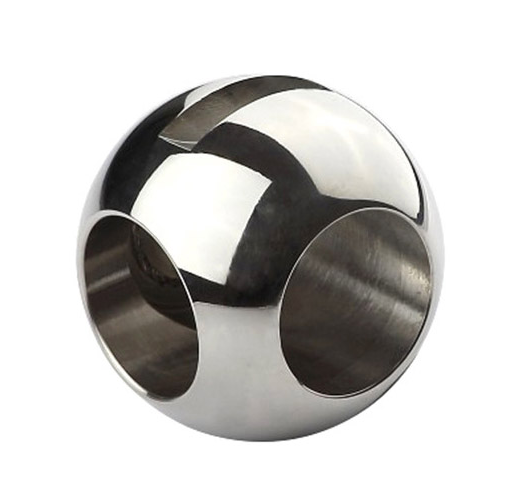S=2(S)2π(^(R^-x^))dx|(0,R)

=4π(S)(^(R^-x^))dx|(0,R)

=4πx^|(0,R)

=4πR^S(k)=根号[R^-(kR/n)^]*2πR/n=2πR^*根号[1/n^-(k/n^)^]

2πR^乘以2就是整个球的表面积4πR^